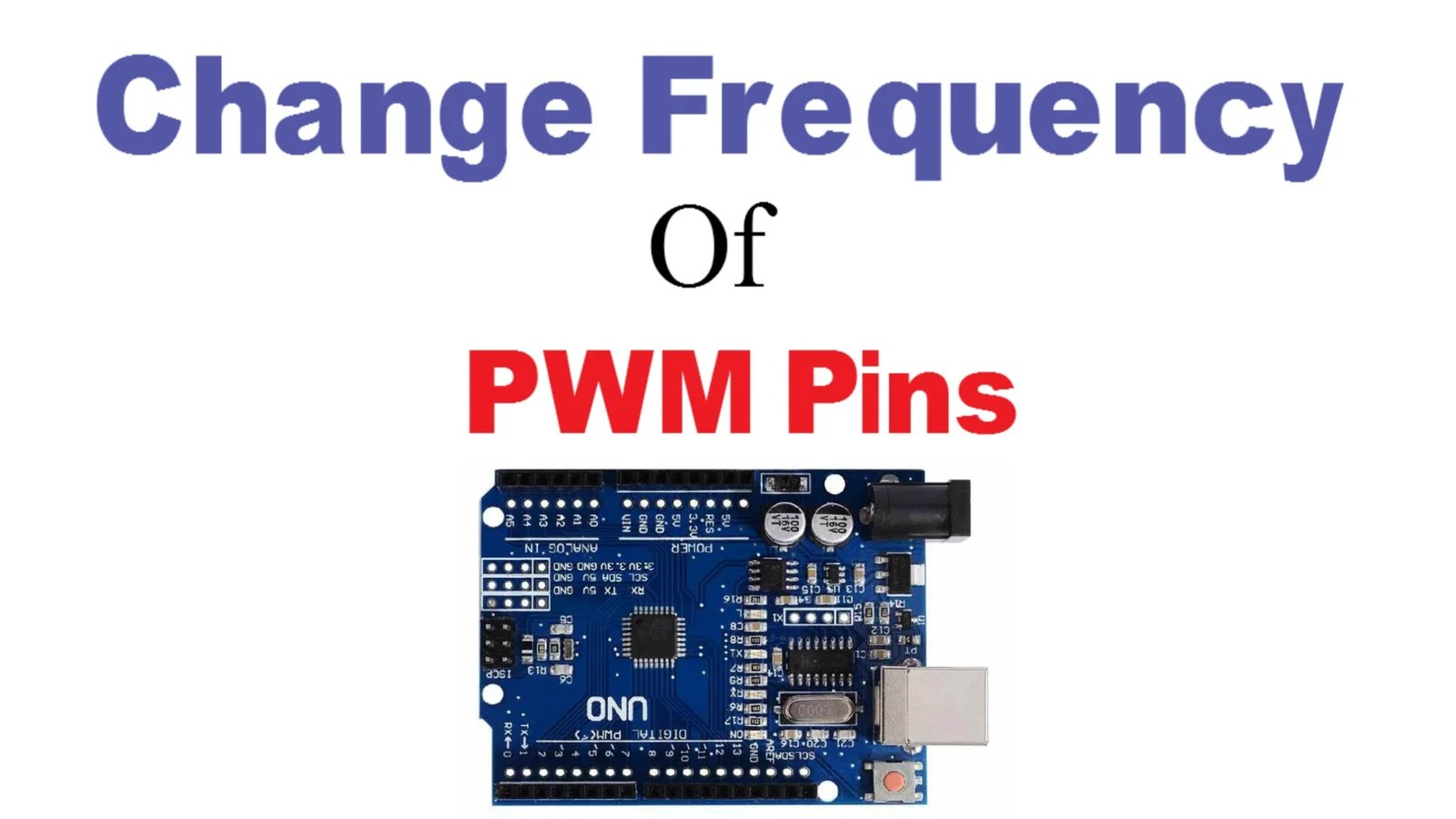# How To Change Frequency On PWM Pins Of Arduino UNO

Arduino Uno is one of the most commonly used Development board these days. It can be used for almost any application. One such application is in high-frequency circuits.

Now there are many facts about Arduino with which many students are not familiar. And one of the facts is this: ” There is a certain default frequency for each PWM pin, which is called when the analogWrite command is used on that pin. And this default frequency can be changed to a value as high as 65Khz and as low as 30Hz by using just one line code”.

 Looking for Arduino Mega PWM frequency change? How To Change Frequency On PWM Pins Of Arduino Mega

You can also watch this video for quick reference:

Here is the default frequency of each PWM pin of Arduino UNO:

PWM frequency for D3 & D11:

490.20 Hz (The DEFAULT)

PWM frequency for D5 & D6:

976.56 Hz (The DEFAULT)

PWM frequency for D9 & D10:

490.20 Hz (The DEFAULT)

Now, these frequencies are optimum for low-frequency applications like fading an LED. But these default frequencies are not suitable for High-frequency circuits. For example, 1Khz is nothing when it comes to an S.M.P.S.

There are many projects in which we require high-frequency pulses, One such project is a Buck-Converter. So to achieve frequency lower or higher than the default frequency on PWM pins, One line code we can use before initializing PWM pin as output is given below:

FOR ARDUINO UNO:

 Code for Available PWM frequency for D3 & D11: TCCR2B = TCCR2B & B11111000 | B00000001; // for PWM frequency of 31372.55 Hz TCCR2B = TCCR2B & B11111000 | B00000010; // for PWM frequency of 3921.16 Hz TCCR2B = TCCR2B & B11111000 | B00000011; // for PWM frequency of 980.39 Hz TCCR2B = TCCR2B & B11111000 | B00000100; // for PWM frequency of 490.20 Hz (The DEFAULT) TCCR2B = TCCR2B & B11111000 | B00000101; // for PWM frequency of 245.10 Hz TCCR2B = TCCR2B & B11111000 | B00000110; // for PWM frequency of 122.55 Hz TCCR2B = TCCR2B & B11111000 | B00000111; // for PWM frequency of 30.64 Hz

 Code for Available PWM frequency for D5 & D6: TCCR0B = TCCR0B & B11111000 | B00000001; // for PWM frequency of 62500.00 Hz TCCR0B = TCCR0B & B11111000 | B00000010; // for PWM frequency of 7812.50 Hz TCCR0B = TCCR0B & B11111000 | B00000011; // for PWM frequency of 976.56 Hz (The DEFAULT) TCCR0B = TCCR0B & B11111000 | B00000100; // for PWM frequency of 244.14 Hz TCCR0B = TCCR0B & B11111000 | B00000101; // for PWM frequency of 61.04 Hz

 Code for Available PWM frequency for D9 & D10: TCCR1B = TCCR1B & B11111000 | B00000001; // set timer 1 divisor to 1 for PWM frequency of 31372.55 Hz TCCR1B = TCCR1B & B11111000 | B00000010; // for PWM frequency of 3921.16 Hz TCCR1B = TCCR1B & B11111000 | B00000011; // for PWM frequency of 490.20 Hz (The DEFAULT) TCCR1B = TCCR1B & B11111000 | B00000100; // for PWM frequency of 122.55 Hz TCCR1B = TCCR1B & B11111000 | B00000101; // for PWM frequency of 30.64 Hz

EXAMPLE:

To show you how frequency changes on applying the above code, Arduino circuit is simulated in Proteus:

1.Two Arduino are selected and placed on Front-Panel

2.Digital Pin 3 ( PWM pin) of each Arduino is connected to oscilloscope

3. Two separate programs are written for each Arduino:

 Program A – Default frequency on Pin 3 void setup() { pinMode(3,OUTPUT); // put your setup code here, to run once: } void loop() { analogWrite(3,155); // put your main code here, to run repeatedly: }

 Program B – Changed frequency on Pin 3 void setup() { TCCR2B = TCCR2B & B11111000 | B00000001; // for PWM frequency of 31372.55 Hz pinMode(3,OUTPUT); // put your setup code here, to run once: } void loop() { analogWrite(3,155); // put your main code here, to run repeatedly: }

4 .Hex file of above programs are given to Arduino

5. Run Simulation6. It can be clearly seen in oscilloscope that frequency is increased to a very high value when this piece of code is used :
TCCR2B = TCCR2B & B11111000 | B00000001; // for PWM frequency of 31372.55 Hz